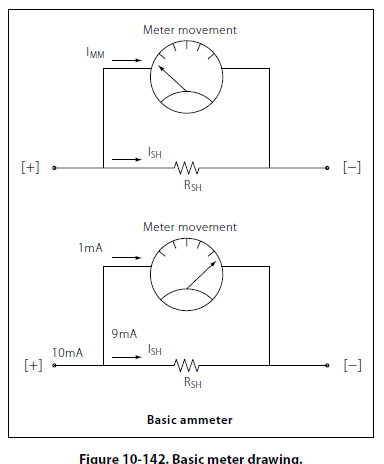D’Arsonval Meter Movement This basic DC type of meter movement — first employed by the French scientist, d’Arsonval in making electrical measurement — is a current measuring device, which is used in the ammeter, voltmeter, and ohmmeter. The pointer is deflected in proportion to the amount of current through the coil. Basically, both the ammeter and the voltmeter are current measuring instruments, the principal difference being the method in which they are connected in a circuit. While an ohmmeter is also basically a current measuring instrument, it differs from the ammeter and voltmeter in that it provides its own source (self-excited) of power and contains other auxiliary circuits. Current Sensitivity and Resistance The current sensitivity of a meter movement is the amount of current required to drive the meter movement to a full-scale deflection. A simple example would be a meter movement that has 1mA sensitivity. What this indicates is that meter movement will require 1mA of current to move the needle to a full-scale indication. Likewise a half scale deflection will require only 0.5mA of current. Additionally, what is called movement resistance is the actual DC resistance of the wire used to construct the meter coil. In a standard d’Arsonval meter movement may have a current sensitivity of 1mA and a resistance of 50O. If the meter is going to be used to measure more than 1mA then additional circuitry will be required to accomplish the task. This additional circuitry is a simple shunt resistor. The purpose of the shunt resistor is to bypass current that exceeds the 1mA limitation of the meter movement. To illustrate this, assume that the 1mA meter in question is needed to measure 10mA. The shunt resistor used should carry 9mA while the remaining 1mA is allowed to pass through the meter. [Figure 10-142]To determine the proper shunt resistance for this situation: RSH = Shunt resistance RM = Meter resistance = 50O Because the shunt resistance and the 50O meter resistance are in parallel, the voltage drop across both of them is the same. ESH = EM Using Ohm’s law, this relationship can be rewritten as: ESH = ISH × RSH EM = IM × RM ISH × RSH = IM × RM Simply solve for RSH RSH =IM × RM/ISH Substituting the values RSH = ImA × 50O/9mA = 5.56O
 ©AvStop Online Magazine                                                                                                                                                      Contact Us              Return To Books